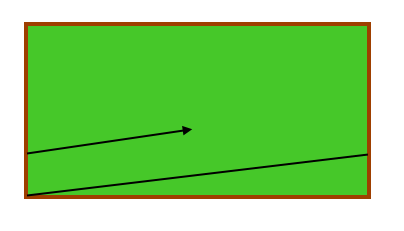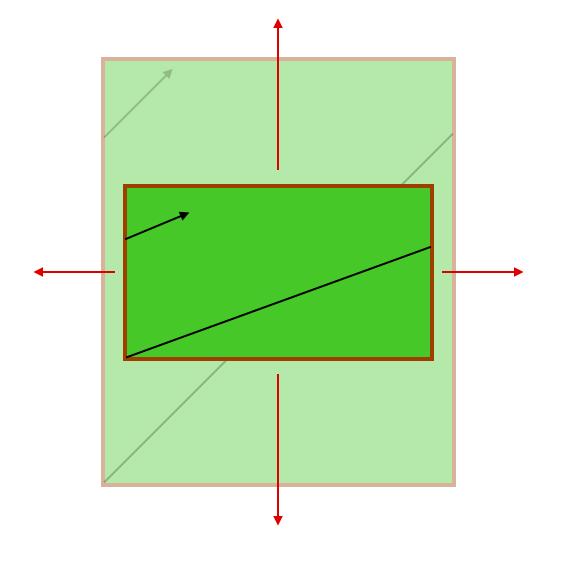## The Billiard Ball Problem

2017 June 3

This problem comes from @mtheorylord on Mathematics Stack Exchange, and I found it very interesting:

Say I have a billiard ball and I launch it at a $45^{\circ}$ angle from the bottom-left corner of a table with length $x$ and width $y$, and it always bounces off of the edges at at $45^{\circ}$ angle. Given $x$ and $y$, will the ball reach a corner again, which corner, and how many times will it need to bounce off the edge?

This problem can be transformed into something less intimidating by noticing that, because of the rotational and reflectional symmetries of the rectangle, we may rotate or reflect the board, shoot out of any corner we like, or even dilate the board, and the number of bounces before entering a corner will remain the same. Let us now assume that $x \ge y$, and superimpose our billiards table into a coordinate plane so that the corner out of which we shoot is at the origin $(0,0)$. The other corners are at $(x,0)$, $(0,y)$, and $(x,y)$.

Because of the properties of a rectangle, we can do the following. Instead of having our ball bounce off of the left and right sides of the billiards table, we can have it "wrap around" the table so that when it hits the right side, it teleports over to the left and continues on its path. We are allowed to do this without altering the number of bounces because this is analogous to reflecting the table and ball whenever the ball hits an edge.Since the ball "wraps around" the table, we can use modular arithmetic to describe its position. Since $x \ge y$ and the ball is shot at a $45^\circ$ angle, the ball will strike the top or bottom after travelling $y$ units horizontally. Thus it has travelled $yn$ units horizontally at the nth bounce. However, because of the "portals" on the sides of the table, its $x$-coordinate is not $yn$ at the nth bounce... instead, it would be

Because whenever its $x$-position reaches $x$, it is reset back to zero again.

When the ball reaches a corner, it will strike the top or bottom whilst its $x$-position is $x$, or when

or when $yn$ is divisible by $x$. Let $n_h$ be the smallest integer $n$ for which this is true. Then we have that

This, then, is the total number of bounces on the top or bottom, including when the ball enters the corner. Now we must find the number of side bounces, or the number of times the ball "wrapped around" the table. We can think of $yn_h$ as the "total horizontal distance" travelled by the ball, and since the ball was teleported after every $x$ units travelled horizontally, there would be a total of

side-bounces. Now, to find the total number of vertical bounces, we must add the number of vertical bounces and horizontal bounces and subtract two (since we counted the entrance of the ball into the corner twice). Then we have

which gives us the number of bounces, not including when the ball enters the corner. Our assumption that $x \ge y$ is no longer important, since we see now that they are interchangable in the formula.

Now what would happen if we shot the ball with a slope of \frac{a}{b}?

Remember what I said earlier about dilating the board? Well, that will become useful in this case. If we have the path of the ball moving at a slope of $\frac{a}{b}$, it is as if we horizontally compressed the line drawing its path by a factor of $a$ and vertically compressed it by a factor of $b$. This means that if we "undo" these compressions by stretching the board and the path, we will have another perfectly straight $45^\circ$ path but with a board of a different side.

When we stretch the board, instead of side lengths of $x$ and $y$, the side lengths will be $ax$ and $by$.Wonderful! We have reduced this to a problem solvable by our previous formula. Since the new side lengths are $ax$ and $by$, the number of bounces is

Now, we have based all of our conclusions on the fact that this billiards table has no side pockets. I will not extend the result to billiards tables with side pockets in this post, but perhaps in a later one.